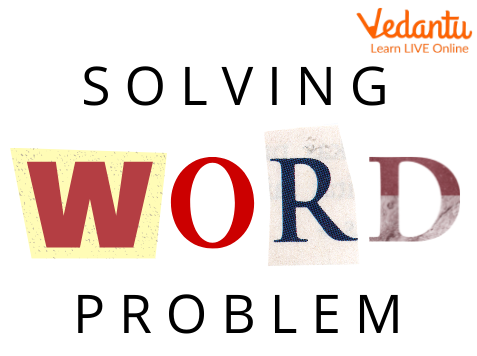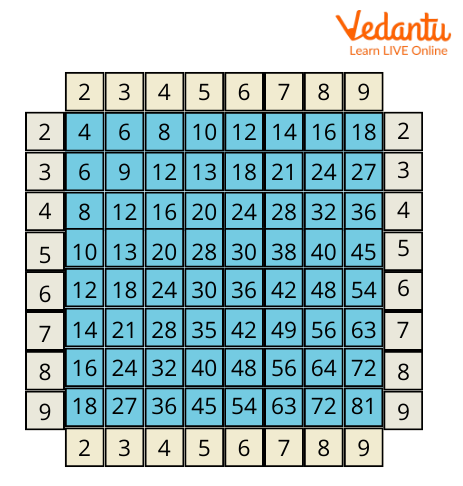Courses
Courses for Kids
Free study material
Free LIVE classes
More

# Multiplication Word ProblemsLIVE
Join Vedantu’s FREE Mastercalss

## Introduction to Multiplication Words Problems

Multiplication Word Problems are multiplication problems that are usually presented in the form of a sentence that generally relates to a real-life scenario.Introduction to Multiplication Word Problems

These word problems will usually be given in a real-life context and will also include some introduction or starting lines to make the question clear.

We will need to read and understand these questions carefully in order to solve the questions. Children can practice multiplication word problems pdf.

Some of the examples of multiplication word problems are listed below:

• In a garden, there is space for 11 rows of 30 seeds in a flower bed. How many seeds are in each flower bed?

• 20 marbles are sorted into boxes of ten. How many boxes are needed?

## Multiplication SumsMultiplication Square

Multiplication is a way through which we can make the process of addition easier.

Suppose we have to add a number several times continuously, like 2+2+2+2+2=10.

But on the other hand, we can just multiply 2 by 5, which will also give us 10.

We can see that the second case is a much simpler and easier way.

This is what we mean by multiplication sums-

Instead of adding the number several times, we can just multiply the number.

Step-by-step solution for the multiplication of two double-digit numbers.

Let us multiply $34 \times 12$.

Step 1: Place the multiplicand (34) on top and the multiplier (12) below it. Multiply the multiplier's one digit by the multiplicand.This results in $34 \times 2=68$. This is the first incomplete item to be displayed in one line.

Step 2: Multiply the multiplicand by the multiplier's tens digit. Here, the multiplicand is 34, and the multiplier's tens digit is 1 . $(34+1)=34$ will be the result.

It should be observed that before writing the second partial product, we must place a zero below the one digit of the partial product. Thus, we arrive at 340.

Step 3: Combine the two partial goods to obtain the complete item. 68 plus 340 will equal $408 .$

## Multiplication Word Problems with Answers

Here are some multiplication word problems with answers-

Q1. How many sheets of paper are there altogether if 20 folders each have 10 sheets of paper inside them?

Ans: Here, either we can add 10 sheets 20 times

Or

Since multiplication is repeated addition, we can also multiply 10 and 20 to get the answer.

$10 \times 20=200$ sheets of paper

Therefore, altogether we have 200 sheets of paper.

Q2. A carton holds 15 packets of biscuits. Each packet has 5 biscuits. How many biscuits can be packed in 10 cartons?

Ans: Here, we'll first find the number of biscuits in one carton-

In one carton, we can pack $15 \times 5$ biscuits.

So in 10 cartons, we can pack $15 \times 5 \times 10$ biscuits.

$15 \times 5 \times 10=750$ biscuits

Therefore, 750 biscuits can be packed in 10 cartons.

## Simple Multiplication Word Problems

Here are some simple multiplication word problems to practice from. One can refer to multiplication word problems pdf to practice too.

Q1. Andrew decided to throw a party and invited some friends. If he has 5 friends coming over and he made 4 sandwiches for each one of them, how many sandwiches did he make in total?

Ans: 20 sandwiches

Q2. Sunil and his 4 friends are deciding on the games that they want to play on his birthday. If each game they decided on takes 10 minutes to end and they prepared a total of 4 games, in how much time will they be able to finish all the games they decided to play?

Ans: 40 minutes

## Summary

Multiplication is a way through which we can easily solve the problem where the same number is being added multiple times.

While attempting multiplication word problems, we need to read the question carefully, understand which number is being multiplied how many times, and then attempt the answer.

Last updated date: 25th Sep 2023
Total views: 125.1k
Views today: 1.25k

## FAQs on Multiplication Word Problems

1. If there are 5 apples in each box then how many apples will be there in 15 boxes?

After reading this question very carefully, we can understand that -

1. There are 5 apples in each box, and

2. We have to find the number of apples in 15 boxes.

In this case, we can either add 5 fifteen times in order to find the number of apples in fifteen boxes.

OR

We can just simply multiply 5 by 15.

In both cases, we will get the answer as 75.

We can also see that the second way (multiplication) is much easier than the first one (addition).

Therefore, there are 75 apples in 15 boxes.

2. A man has 3 oranges. If he cuts each orange into 2 parts, how many pieces of orange will he have?

After reading this question very carefully, we can understand that -

1. 3 oranges have been divided into 2 parts each.

2. We have to find the number of pieces of orange.

In this case, we can either add 2 three times to find the total pieces of orange.

OR

We can just simply multiply 3 by 2.

In both cases, we’ll get the answer as 6 pieces.

We can also see that the second way (multiplication) is much easier than the first one (addition).

Therefore, he will have 6 pieces of orange.